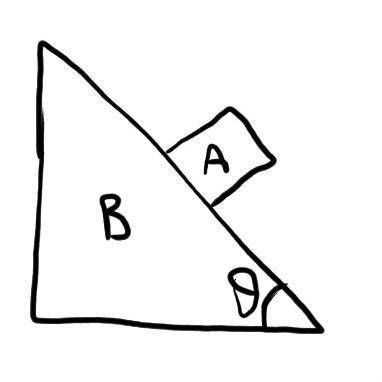# Newton's Laws of Motion Problem

## Homework Statement

Mass of Block A = 1 kg
Mass of Wedge B = 2 kg
Inclination of Wedge is 37°
and Cos 37° = 4/5
The wedge is given an acceleration of 5 ms-2
Find -
1. Acceleration of Block wrt Wedge
2. Normal Force## Homework Equations

Newton's Law for a System (hereby referred as NLS)
∑fext = m1a1+m2a2......+mnan

## The Attempt at a Solution

Let the Acceleration of Block wrt Wedge be λ along the inclination.
In Y axis, there is only one Ext force i.e. The gravity and ∑fnet in Y direction is m1g+ m2g = (m1+ m2)g
Therefore in Y axis using Newton's Law for a System,
mAaA + mBaB = (m1+ m2)g
but in Y axis , aA = 0
and ab in Y axis = λsinθ + aAcos 90
= λsinθ + 0
= λsinθ
Therefore, mBaB = (m1+ m2)g
1 (λsin(θ)) = (1 + 2)g
λ sin 37° = (3)10
λ(3/5) = 30
λ = 50
but using pseudo force, λ = 2 ms-2 which is correct answer in the Answer Key
Where am I going Wrong?

#### Attachments

Is wedge B being given an acceleration horizontally to the left or right?

Assuming then no friction block A will also be sliding down the inclined wedge B.

haruspex